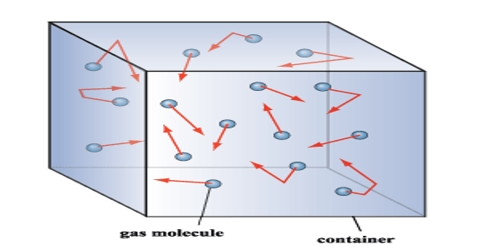# Kinetic theory of Gases

### Kinetic Theory:

To explain the physical properties of the gases, different scientist give different opinion. But, the ideas which are accepted are known as Kinetic Theory of gases.

Postulates of Kinetic Theory of Gases:

(i) Formation of gases: A gas is composed of a large number of minute, discrete, spherical particles of identical mass and size. The particles are called the molecules.

(ii) Volume: The volume occupied by the gaseous molecules is negligible as compared to the total volume occupied by the gas.

(iii) Mobility of molecules: There being no intermolecular forces of attraction or repulsion between the molecules, they are in a continuous random movement in all possible directions and they move in a straight line.

(iv) Elasticity of motion of molecules: While moving, the molecules collide with each other and on the walls of the container. During these collisions they change their directions of motion without any loss in their Kinetic energy i.e. the collisions are perfectly elastic.

(v) Pressure: The pressure exerted by the gas is due to the collisions of the gas molecules on the walls of the container. The large the number of collisions, the greater is the pressure of the gas.

(vi) Kinetic energy: The average Kinetic energy of a gas molecule is directly proportional to the absolute temperature of the gas.

(vii) Effect of gravitational force: The pressure exerted by the gas does not depend on the gravitational force of attraction.

(viii) Mean free path: The average distance between two successive collision is called mean free path.

(ix) Collision time: The collision time with respect to the time between two successive collision is negligible.

Equation for kinetic Theory of Gas:

From the above postulates a kinetic equation for ideal gases is deduced which is given below-

PV = 1/3 mNc2; where, P=Pressure of all gases

V=Volume of the container of gas
N=Number of molecules of gas
m=mass of each molecules